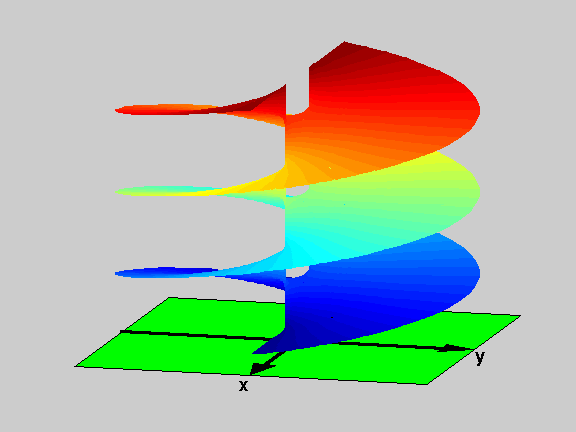18.04 | Fall 1999 | Undergraduate

# Complex Variables with Applications

Study Materials

## Riemann Surfaces: f(z)=log(z2-1) View 1

(All images created with MATLAB® software)

Riemann Surface for f(z)=log(z2-1). The picture shows only three levels of the surface, which extends up and down with infinitely many similar levels (again, notice the similarity with a parking building). This is an example with three branch points, which are at: z=1, z=-1 and infinity (in all the prior examples there were only two branch points). Geometrically, using the analogy with a parking building, we note that here the connecting ramps are actually two, one going from infinity to z=-1 and the other from z=1 to infinity.

Picture obtained using the script RiemannSur in the Athena 18.04 MATLAB® Toolkit.Figure 1: Riemann Surface of the function f(z)=log(z2-1)